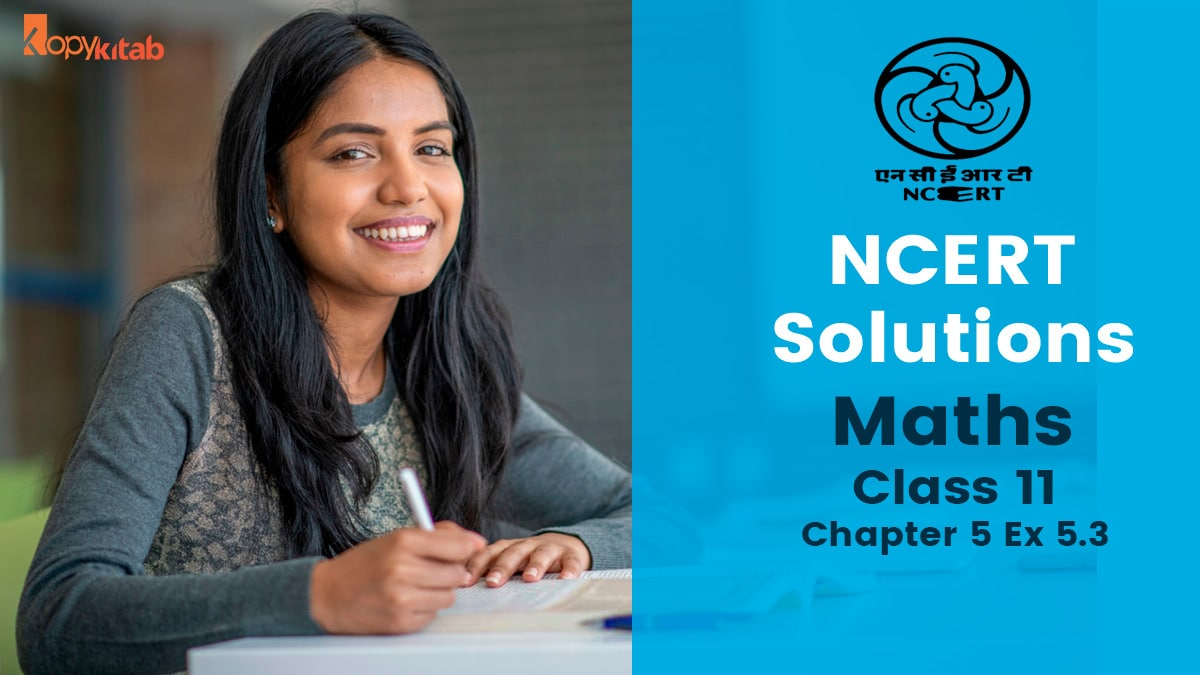# NCERT Solutions Class 11 Maths Chapter 5 Complex Numbers and Quadratic Equations Exercise 5.3 (2021-22)

###NCERT Solutions Class 11 Maths Chapter 5 Exercise 5.3 :To learn how to solve problems, students need first have an understanding of how the problems in the exercises are solved. Understanding the notion is required to grasp the problem-solving method. Quadratic Equations are the basis of Exercise 5.3 of NCERT Solutions for Class 11 Maths Chapter 5 – Complex Numbers and Quadratic Equations. A quadratic equation in algebra is any equation of the form ax2+bx+c=0, where x is unknown and a, b, and c are known values, with a 0.

Solving the issues in this exercise helps students understand how to solve a problem involving quadratic equations. The activity consists of ten questions that students can answer and practise. For a better comprehension of the ideas, they might consult the NCERT Solutions for Class 11 Maths.

## NCERT Solutions Class 11 Maths Chapter 5 Exercise 5.3

NCERT Solutions for Class 11 Maths Chapter 5 Ex 5.3

### Download NCERT Solutions Class 11 Maths Chapter 5 Exercise 5.3 Free PDF

Exercise 5.3 Class 11 Maths NCERT Solutions Free PDF

The subject matter experts on staff methodically create the NCERT solutions. On kopykitab, you can view and download NCERT solutions for class 11.

## Access NCERT Solutions Class 11 Maths Chapter 5 Ex 5.3

Ex 5.3 Class 11 Question 1:Ans:Ex 5.3 Class 11 Question 2:Ans:ch 5 Maths Class 11 Ex 5.3 Question 3:Ans:ch 5 Maths Class 11 Ex 5.3 Question 4:Ans:ch 5 Maths Class 11 Ex 5.3 Question 5:Ans:ch 5 Maths Class 11 Ex 5.3 Question 6:Ans:ch 5 Maths Class 11 Ex 5.3 Question 7:Ans:ch 5 Maths Class 11 Ex 5.3 Question 8:Ans:ch 5 Maths Class 11 Ex 5.3 Question 9:Ans:ch 5 Maths Class 11 Ex 5.3 Question 10:Ans:We have covered everything related to ” CBSE NCERT Solutions Class 11 Maths Chapter 5 Exercise 5.3″. If you have any doubts, feel free to ask any queries in the comment section below.

## FAQs on NCERT Solutions Class 11 Maths Chapter 5 Exercise 5.3

Here are the most frequently asked questions related to NCERT Solutions Class 11 Maths Chapter 5 Exercise 5.3 by the students.

### Where can I get Class 11 Maths NCERT Solutions for Chapter 5 Exercise 5.3?

You can get the NCERT Solutions for Class 11 Maths Chapter 5 Exercise 5.3 from the pdf given in the article above.

### How many exercises are there in Chapter 5 of Maths NCERT of Class 11?

There are a total of 3 exercises and one miscellaneous exercise in chapter 5 of Maths NCERT of Class 11.

### What are Class 11 NCERT Maths Solutions?

Based on the CBSE board’s most recent rules, NCERT experts have produced solutions. All chapters from the CBSE Maths Textbooks for the 11th grade are included.

### What are the benefits of Maths NCERT Solutions?

The benefits of Maths NCERT Solutions is that while studying the issue, you can learn the proper response and use it to guide you in the right way. It will help you save time.

### What is Chapter 5 of NCERT Maths of Class 11?

Chapter 5 of NCERT Maths of Class 11 is Complex Numbers and Quadratic Equations.

### What is the exercise 5.3 of the NCERT Maths of Class 11 Chapter 5 based on?

Quadratic Equations are the basis of Exercise 5.3 of NCERT Solutions for Class 11 Maths Chapter 5- Complex Numbers and Quadratic Equations. A quadratic equation in algebra is any equation of the form ax2+bx+c=0, where x is unknown and a, b, and c are known values, with a 0.

Effective And Quick Preparation For CET How to ace in Multiple Choice Questions (MCQ) exams Avoid These Mistakes While Studying For Board Exams The Importance Of Learning Basic Life Skills 6 Ways To Make Social Science Your Favourite Subject